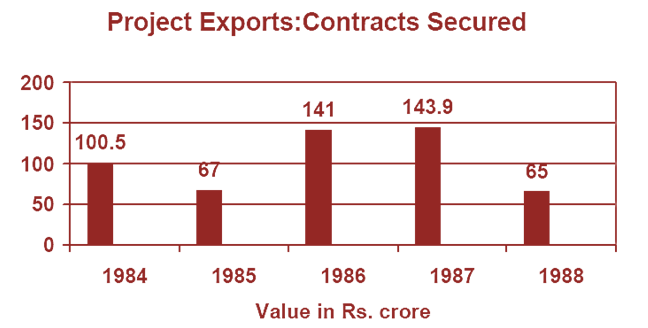Direction for the question: Refer to the following Bar-chart and answer the questions that follow:Question 1: What is the average value of the contract secured during the years shown in the diagram?
(a) Rs. 103.48 crore
(b) Rs. 105 crore
(c) Rs. 100 crore
(d) Rs.125.2 crore

Question 2: Compared to the performance in 1985 (i.e. taking it as the base), what can you say about the performances in the years ’84, ’85, ’86, ’87, ’88 respectively, in percentage terms?
(a) 150, 100, 211, 216, 97
(b) 100, 67, 141, 144, 65
(c) 150, 100, 200, 215, 100
(d) 120, 100, 220, 230, 68

Question 3: Which is the year in which the highest percentage decline is seen in the value of contract secured compared to the preceding year?
(a) 1985
(b) 1988
(c) 1984
(d) 1986

This is a simple question. We simply need to find the average value in this case. This value can be calculated as follows:

(100.5 + 67 + 141 + 143.9 + 65)/5 = 103.48